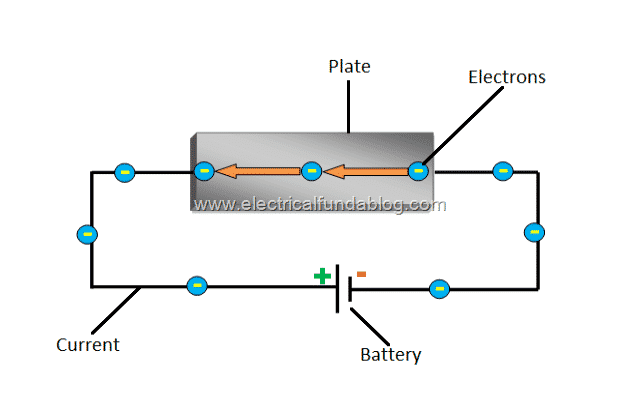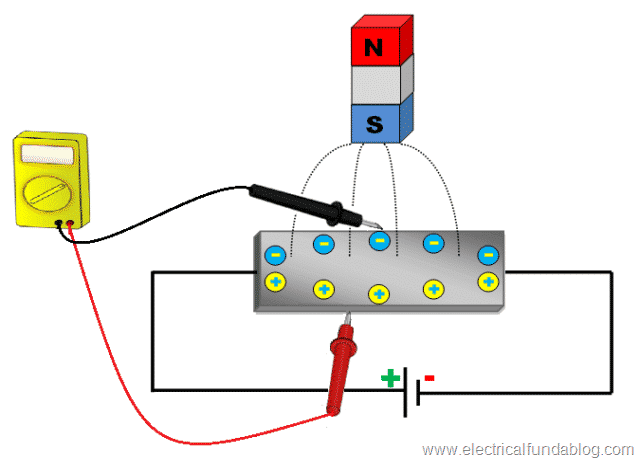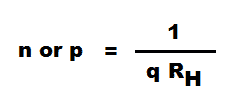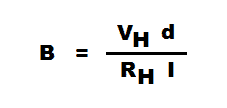Saturday, December 9, 2023
HomePower ElectronicsHall EffectHall Effect Principle - History, Theory Explanation, Mathematical Expressions and Applications

# Hall Effect Principle – History, Theory Explanation, Mathematical Expressions and Applications

Hall Effect Principle is one of the most popular theories in magnetic field measurement. This post will discuss Hall Effect Principle, its history, theory explanation, applications and mathematical expressions of Hall Effect Principle including calculations for Hall Voltage, Hall Coefficient, Charge Carrier Concentration, Hall Mobility and Magnetic Field Density.

Hall Effect Principle explains the behavior of charge carriers when it is exposed to electricity and magnetic fields. This principle can be regarded as an extension to Lorentz Force which is the force acting on the charge carriers (Electrons and Holes) passing through a magnetic field.

The sensors working on this Principle are called Hall Effect Sensors. These Hall Effect Sensors are in high demand and have very wide spread applications such as Proximity sensors, Switches, Wheel speed sensors, Positional sensors, etc.

## History Behind Hall Effect

The Hall Effect Principle has been named after an American physicist Edwin H. Hall (1855–1938). It was first introduced to the world by him in 1879.Fig. 1 – Photo of Edwin H. Hall – Discovered Hall Effect Principle

In 1879, he discovered that when a current carrying conductor/ semiconductor is placed perpendicularly to a magnetic field, a voltage is generated that could be measured at right angles to the current path. During that time, Electric current in a wire was believed something similar to a flowing liquid in a pipe.

Hall Effect Principle suggested that the magnetic force in a current results in a crowding at the end of Pipe or (wire). Electromagnetic principle has now further explained the science behind Hall Effect with a much better appreciation. This Hall’s theory was definitely well ahead of its time. Only two decades later, with the introduction of semi-conductors, the work and uses of Hall Effect was utilized in an effective manner.

Initially, this principle was used for Chemical sample classification. Later on, Hall Effect Sensors (with the use of Indium Arsenide semiconductor compounds) came as a source to measure DC or Static magnetic fields without keeping the sensor in motion. After a decade in 1960s, silicon based semiconductors came into existence. It was the time when Hall elements were combined with integrated amplifiers and so Hall switch was introduced to the world.Fig. 2 – Hall Effect Principle – Current Flowing Through a Plate

## Hall Effect Principle

Hall Effect Principle says that when a conductor or semiconductor with current flowing in one direction is introduced perpendicular to a magnetic field a voltage could be measured at right angles to the current path.

The effect of getting a measurable voltage, as told above, is called the Hall Effect.

## Theory Behind Hall Effect Principle

Before anything, we should understand what actually Electric current is. Electric Current is basically a flow of charged particles through a conductive path. These charged particles may be ‘Negative Charged Electrons’ or even ‘Positive Charged Holes’ (voids where electrons should be). Now Let’s come to the topic.

If we take a thin conductive plate (as shown above in Fig 1 and reiterated below for ease of reader) and connect it in a circuit with a battery (voltage source) then a current will start flowing it. The charge carriers will flow in a straight line from one end of the plate to the other end.Fig. 2 – Hall Effect Principle – Current Flowing Through a Plate

As the charge carriers are in motion, they will produce a magnetic field. Now when you place a magnet near the plate, its magnetic field will distort the magnetic field of the charge carriers. This will upset the straight flow of the charge carriers. The force which upsets the direction of flow of charge carriers is known as Lorentz force.

Due to the distortion in the magnetic field of the charge carriers, the negative charged electrons will be deflected to one side of the plate and positive charged holes to the other side. That is why a potential difference (also called as Hall Voltage) will generate between both sides of the plate which can be measured using a meter.Fig. 3 – Hall Effect Principle – Deflection of Electrons and Holes

This effect is known as Hall Effect. The stronger the magnetic field, the more the electrons will be deflected. This means that the higher the current, the more the electrons will be deflected. And, the more the electrons will be deflected, the more the potential difference will be observed between both sides of the plate. Therefore we can say that:

• Hall Voltage is directly proportional to Electric Current, and
• Hall Voltage is directly proportional to the applied magnetic field.

## Mathematical Expressions for Hall Effect Principle

Here are some mathematical expressions which are widely used in Hall Effect calculations: –

### Hall Voltage

Hall Voltage is represented by VH. The mathematical Expression for Hall voltage is:-Where:

I – Current flowing through the Sensor

B – Magnetic Field Strength

q – Charge

n – number of charge carriers per unit volume

d – Thickness of the Sensor

### Hall Coefficient

It is represented by RH. Mathematical expression for Hall Coefficient (RH) is 1/(qn). The Hall Coefficient (RH) is positive if the number of positive charge Holes are more than the number of negative charge Electrons. Similarly, The Hall Coefficient (RH) is negative if the number of negative charge Electrons are more than the number of positive charge Holes.

### Charge Carrier Concentration

The Charge Carrier Concentration of Electrons is represented by ‘n’ and Holes is represented by ‘p’. The mathematical expression for the Charge Carrier Concentration is: –### Hall Mobility

The Hall Mobility for Electrons is represented by ‘μn‘ and for Holes is represented by ‘μp‘. The mathematical expression for the Hall Mobility is: –Where:

μn – Conductivity due to Electrons

μp – Conductivity due to Holes

### Magnetic Flux Density

The Magnetic Flux Density is represented by ‘B’. The mathematical expression for the Magnetic Flux Density is: –## Applications of Hall Effect Principle

Hall Effect Principle is used in: –

• Magnetic field sensing equipment.
• Multiplier applications to provide actual multiplications.
• Hall Effect Tong Tester for measurement of direct current.
• Phase angle measurement. For Example – in measuring angular position of the crank shaft to accurately align with the firing angle of the spark plugs
• Linear or Angular displacement transducers. For example – to identify the position of the car seats and seat belts and act as an interlock for air-bag control.
• Proximity detectors.
• Hall Effect Sensors and Probes
• For detecting wheel speed and accordingly assist anti-lock braking system (ABS).

## How Hall effect can be used to identify type of Semiconductor used?

The Hall Coefficient tells it all. If the Hall Coefficient is negative, it means that the majority charge carriers are Electrons. And as the number of electrons are more compared to Holes in n-type semiconductors, that clearly indicates that the semiconductor being tested is n-type. Similarly, if the Hall Coefficient is positive, it means that the majority charge carriers are Holes. And as the number of Holes are more compared to electrons in p-type semiconductors, that clearly indicates that the semiconductor being tested is p-type.Ratna
Ratna is a B.E (Computer Science) and has work experience in UK Mainframe IT industry. She is also an active Web Designer. She is an author, editor and core partner at Electricalfundablog.
RELATED ARTICLES